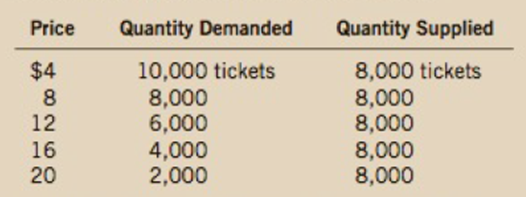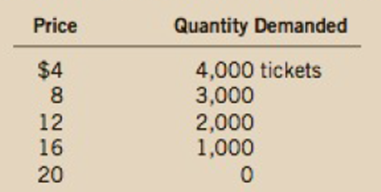Chapter 4, Problem 11PA

Chapter
Section
Textbook Problem

Suppose that the price of basketball tickets at your college is determined by market forces. Currently, the demand and supply schedules are as follows:a. Draw the demand and supply curves. What is unusual about this supply curve? Why might this be true?b. What are the equilibrium price and quantity of tickets?c. Your college plans to increase total enrollment next year by 5,000 students. The additional students will have the following demand schedule:Now add the old demand schedule and the demand schedule for the new students to calculate the new demand schedule for the entire college. What will be the new equilibrium price and quantity?To find additional study resources, visit ccngagebrain.com, and search for “Mankiw.”

Subpart (a):

To determine
Equilibrium in the market.

Explanation

Figure 1 illustrates the equilibrium position in the economy.

Figure 1 illustrates the demand and supply curves of basketball tickets...

Subpart (b):

To determine
Equilibrium in the market.

Subpart (c):

To determine
Equilibrium in the market.

Still sussing out bartleby?

Check out a sample textbook solution.

See a sample solution

The Solution to Your Study Problems

Bartleby provides explanations to thousands of textbook problems written by our experts, many with advanced degrees!

Get Started

Find more solutions based on key concepts